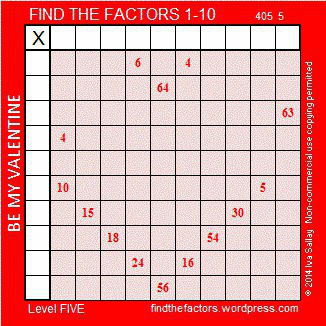# 59 and Valentine Gif

• 59 is a prime number.
• Prime factorization: 59 is prime.
• The exponent of prime number 59 is 1. Adding 1 to that exponent we get (1 + 1) = 2. Therefore 59 has exactly 2 factors.
• Factors of 59: 1, 59
• Factor pairs: 59 = 1 x 59
• 59 has no square factors that allow its square root to be simplified. √59 ≈ 7.6811How do we know that 59 is a prime number? If 59 were not a prime number, then it would be divisible by at least one prime number less than or equal to √59 ≈ 7.7. Since 59 cannot be divided evenly by 2, 3, 5, or 7, we know that 59 is a prime number.

59 is never a clue in the FIND THE FACTORS puzzles.Valentine’s Day is almost here. Here is a Valentine you can share with your friends who enjoy solving puzzles.

To solve the puzzle, the numbers 1 through 10 need to be written on the top row and also on the first column so that those numbers and the clues inside the puzzle make a multiplication table. Since it’s a level 5 puzzle, it may be a little challenging.

Your friend will not have to wait a whole week for the solution because there is a gif that will solve it quickly and logically. The gif could be the valentine, too.

This week’s puzzles are available in an excel file here. If you have a spreadsheet program on your computer, you can access it. If you enable editing in excel, you can type your answers directly onto the puzzle, and you can also easily print the puzzles.

Here are the factors to last week’s puzzle.<a data-pin-do=”embedPin” href=”http://www.pinterest.com/pin/506092076847813688/”></a>
<!– Please call pinit.js only once per page –>
//assets.pinterest.com/js/pinit.js